3.4 Sequential Search of Ordered Array with Sentinel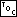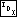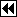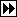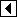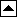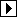When we implemented sequential search in a sorted array, we lost the benefits of having a sentinel. But we can reintroduce a sentinel in the same way we did before, and obtain some of the same benefits. It's pretty clear how to proceed:

```19. <Sequentially search a sorted array of ints using a sentinel 19> =
/* Returns the smallest i such that array[i] == key,    or -1 if key is not in array[].
array[] must be an modifiable array of n ints,    sorted in ascending order,
with room for a (n + 1)th element at the end. */
int seq_sorted_sentinel_search (int array[], int n, int key) {
int *p;

array[n] = key;
for (p = array; *p < key; p++)
/* Nothing to do. */;
return p - array < n && *p == key ? p - array : -1;
}
```

This code is included in 600.

With a bit of additional cleverness we can eliminate one objection to this sentinel approach. Suppose that instead of using the value being searched for as the sentinel value, we used the maximum possible value for the type in question. If we did this, then we could use almost the same code for searching the array.

The advantage of this approach is that there would be no need to modify the array in order to search for different values, because the sentinel is the same value for all searches. This eliminates the potential problem of searching an array in multiple contexts, due to nested searches, threads, or signals, for instance. (In the code below, we will still put the sentinel into the array, because our generic test program won't know to put it in for us in advance, but in real-world code we could avoid the assignment.)

We can easily write code for implementation of this technique:

```20. <Sequentially search a sorted array of ints using a sentinel (2) 20> =
/* Returns the smallest i such that array[i] == key,    or -1 if key is not in array[].
array[] must be an array of n ints,    sorted in ascending order,
with room for an (n + 1)th element to set to INT_MAX. */
int seq_sorted_sentinel_search_2 (int array[], int n, int key) {
int *p;

array[n] = INT_MAX;
for (p = array; *p < key; p++)
/* Nothing to do. */;
return p - array < n && *p == key ? p - array : -1;
}
```

This code is included in 600.

Exercises:

1. When can't the largest possible value for the type be used as a sentinel? [answer]